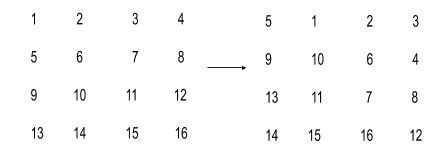# Rotate Matrix | Given a 2-dimensional matrix of size ‘N’ x ‘M’, rotate the elements of the matrix clockwise.

## Rotate Matrix

Problem Statement

#### Given a 2-dimensional matrix of size ‘N’ x ‘M’, rotate the elements of the matrix clockwise.

``````For example:
Input Matrix: [ [ 1, 2, 3 ]
[ 4, 5, 6 ]
[ 7, 8, 9 ] ]

Output Matrix: [ [ 4, 1, 2 ]
[ 7, 5, 3 ]
[ 8, 9, 6 ] ]

The output matrix is generated by rotating the elements of the input matrix in a clockwise direction. Note that every element is rotated only once.
``````
##### Note :
``````You do not need to print anything; it has already been taken care of. Also, update the given matrix in-place.
``````
##### Input Format :
``````The first line of input contains an integer 'T' representing the number of test cases. Then the test cases follow.

The first line of each test case contains two single-spaced integers N and M, representing the number of rows and columns of the matrix, respectively.

The next N line contains M single-spaced integers denoting the matrix elements.
``````
##### Output Format :
``````For each test case, the modified matrix is printed.

The output for each test case is in a separate line.
``````
##### Constraints :
``````1 <= T <= 10
1 <= N, M <= 100
-10^5 <= data <= 10^5,

where ‘T’ is the number of test cases,  ‘N’ and ‘M’ are the numbers of rows and columns respectively and ‘data’ is the value of the elements of the matrix.
``````
##### Sample Input 1 :
``````1
4 4
1 2 3 4
5 6 7 8
9 10 11 12
13 14 15 16
``````
##### Sample Output 1 :
``````5 1 2 3
9 10 6 4
13 11 7 8
14 15 16 12
``````
##### Explanation Of Sample Input 1 :``````The resulting matrix after rotating the given matrix clockwise is shown above.
``````
##### Sample Input 2 :
``````2
2 2
1 3
4 5
3 3
3 4 5
5 6 7
8 10 20
``````
##### Sample Output 2 :
``````4 1
5 3
5 3 4
8 6 5
10 20 7``````

### `Solution to the Above Problem is `

``void rotateMatrix(vector<vector<int>> &mat, int n, int m){    int sr=0,sc=0,er=n-1,ec=m-1;    int pre,curr;    if(n==m & n%2==0){        while(sr<=er && sc<=ec){        if(sr+1<=er)pre=mat[sr+1][sc];        for(int i=sc; i<=ec; i++){            curr=mat[sr][i];            mat[sr][i]=pre;            pre=curr;        }        sr++;        for(int i=sr;i<=er; i++ ){            curr=mat[i][ec];            mat[i][ec]=pre;            pre=curr;        }        ec--;                for(int i=ec; i>=sc; i--){            curr=mat[er][i];            mat[er][i]=pre;            pre=curr;        }        er--;        for(int i=er; i>=sr; i--){            curr=mat[i][sc];            mat[i][sc]=pre;            pre=curr;        }        sc++;    }    }    else{        while(sr<er && sc<ec){        if(sr+1<=er)pre=mat[sr+1][sc];        for(int i=sc; i<=ec; i++){            curr=mat[sr][i];            mat[sr][i]=pre;            pre=curr;        }        sr++;        for(int i=sr;i<=er; i++ ){            curr=mat[i][ec];            mat[i][ec]=pre;            pre=curr;        }        ec--;                for(int i=ec; i>=sc; i--){            curr=mat[er][i];            mat[er][i]=pre;            pre=curr;        }        er--;        for(int i=er; i>=sr; i--){            curr=mat[i][sc];            mat[i][sc]=pre;            pre=curr;        }        sc++;    }    }    }``
`Thank you for reading.`